# Auto model

Member Posts: 4Newbie
in Help
Hi
What are the next steps after I manage to generate a good Auto Model
How I can implement that model to the rest of data and check it performance and ..If there is possibility to connect interactive data ..stock prices... to generate live pricing based on generated model
So basically it is not a new question but I could not figure out those given answers so far
Video or step by step instruction might help
Thank you

• Member Posts: 563Unicorn
edited March 9
Hello @Sony

If you want to use the exact model generated in automodel, you can open the process by selecting one model. In the below screenshot I selected Naive Bayes and then clicked on open process (these are highlighted in red circles below).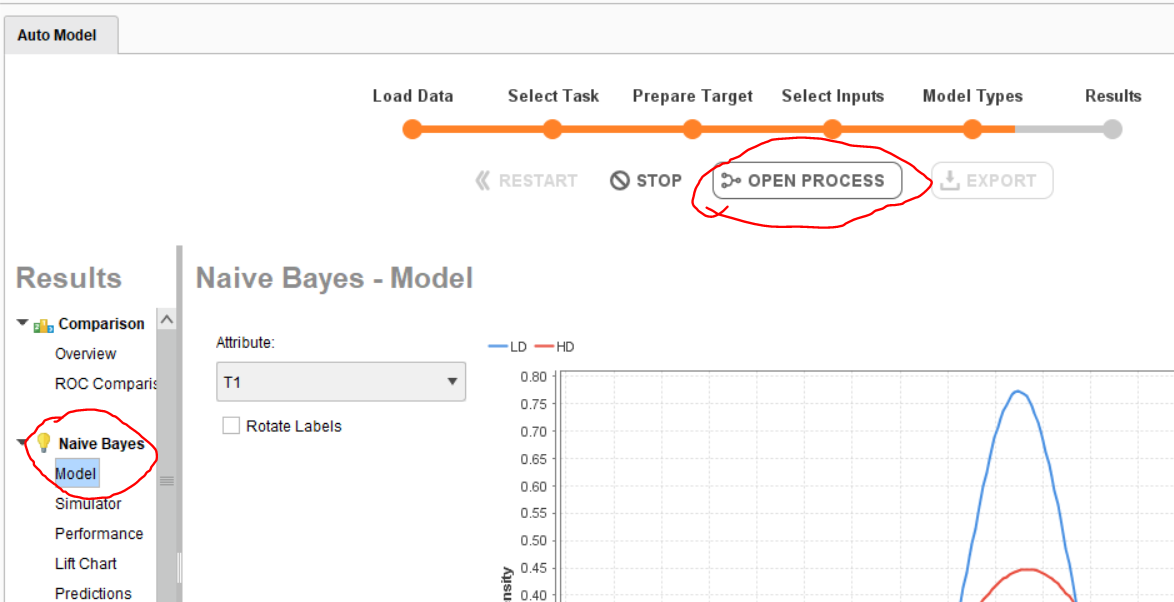One you open this you will find below screen where you can observe all the operators used in this model.  Here you can change or delete sampling operators based on your needs and run the process. You can also change other operators, add different models based on your need.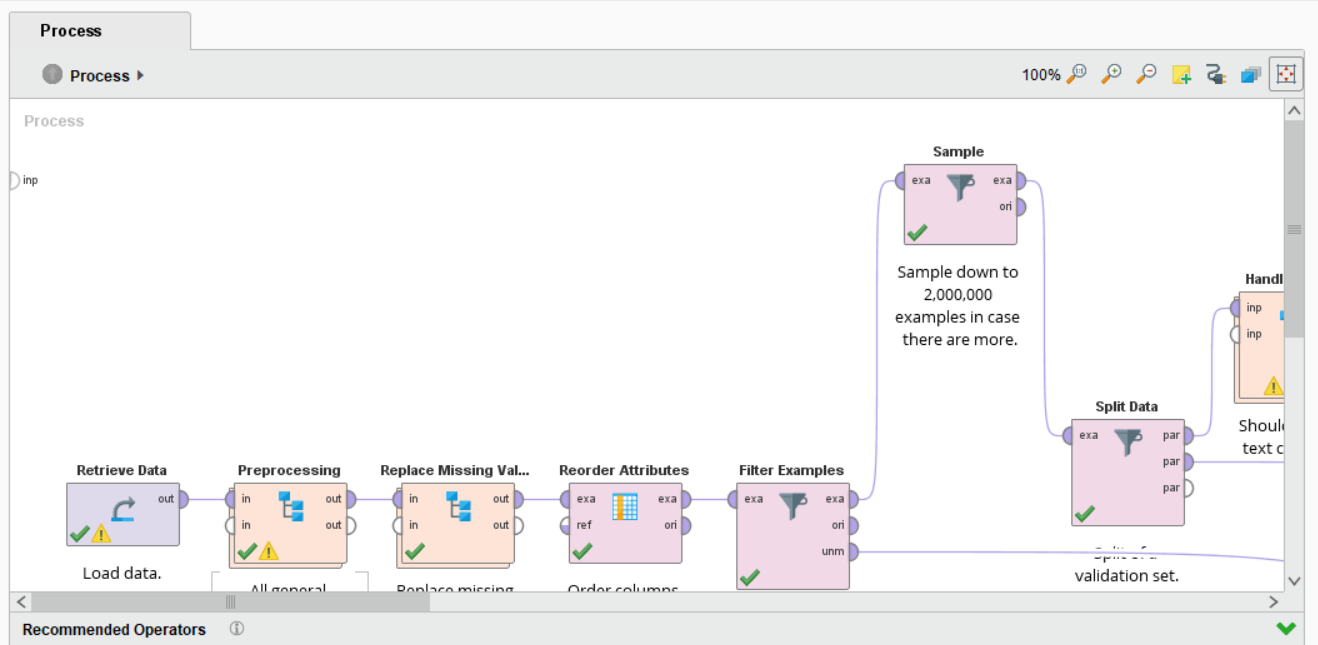For detailed explanation: You can check auto model related videos in the below link
• Member Posts: 4Newbie
Thank you very much Varun ....So Auto Model has to be tested on whole sample later on .?Auto model is just first step in modeling ...?I have to check how it works on the rest of the sample now ...? How it can be done ? After that is checked how I can proceed with using that very same model ? How I can connect it with outsourced data ...I guess it is not planned to go to SIMULATOR and manually input values to get forecast result ....Sorry if i am not very clear but whole idea is how to proceed and use that very model later on

• Administrator, Moderator, Employee, RapidMiner Certified Analyst, RapidMiner Certified Expert, Community Manager, RMResearcher, Member, University Professor Posts: 1,619RM Founder
Hi Sony,
There are three options here:
1. If the scoring is a one time thing, the easiest way is to simply add the data rows to be scored to the input data set for Auto Model - the underlying process will automatically apply the model on those rows.  However, you may want to use the model on a frequent base and without rebuilding it every time.  In this case you will need to follow one of the two procedures below.
2. You take the process created by Auto Model and turn it into a scoring process.  This is most useful if you need to score complete data sets instead of single rows at a time.  After you have opened the process as @varunm1 has described, you add a couple of Store operators wherever you want to store something.  In your case you want to store the model at least.  If you used feature engineering as well, you also want to store the final feature set.  If you did text analytics, you also want to store the text model.  Run this process again and store the model and the other desired results in your repository.
Now change the process and throw pretty much everything away from the model building itself but keep the basic preprocessing in the beginning.  Now Retrieve you stored objects and apply them to the resulting data (after the prep).  First text, then feature set, then the actual prediction model.  This new process can be used now on the the same type of input data for scoring.  You may want to add some storage of the results at the end obviously.
3. You create a simplified scoring process only working on a single row.  This is most useful if you want to turn this into a web service for integration with RapidMiner Server.  Here you can do a much simpler approach.  You start like above (open the process, store the model, run it again).  But this time you start with a single row data set of your model training data (preferably all averages / mode values - I store this as well next to the model and use Aggregate to build the desired values).  Now you can use a chain of Set Data operators to set the desired values one by one.  I typically use macros from the process context to retrieve the desired values.  This way, I can now simply turn this process into a web service which can be integrated.
As of today, option 1 is easy to achieve but may not be applicable.  Options 2 and 3 require a bit more work (but can be still done in 15 minutes or so).  BTW, in one of the next versions of Auto Model there will be a new Deploy function where the procedures for 2 and 3 will be done for you.  I still think is beneficial to learn how to do this so you can customize the deployment to your needs even if the bulk of the work is done for you then.
Cheers,
Ingo
• Member Posts: 4Newbie
Hi Ingo,
If Auto Model require testing on a full sample additionally or model is done and I can just add new rows as you have mentioned in solution No. 1 and start on continuing..
Do you by chance have any kind of video of above mentioned  ?
I apologize but dont know why it doesn't "click me"..maybe missing some brain cells ..lol
Thank you very much
• Member Posts: 4Newbie
Thank you guys
Will wait for that update
Have a nice weekend
• Member Posts: 31Contributor II
edited May 4
@varunm1 / @IngoRM

I'm having issues reusing a model originally created by Auto Model.

I've taken time series data, created features, windows, and a label/horizon, and stored it as a RM data storage object. I fed that data to Auto Model and got these results: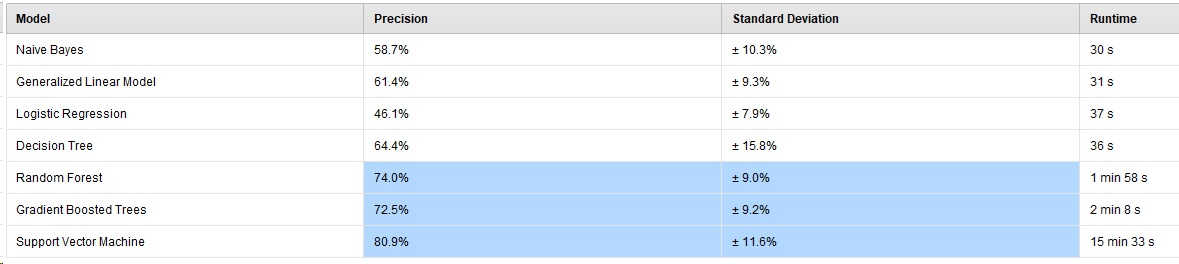I opened the GBT Auto Model process, reran it, and stored the model.

I then took holdout data (periods following the training data’s timeframe), processed and stored it as before, and ran it through the pre-processing steps of the Auto Model process. I retrieved the GBT model and passed it, along with the holdout data, to Apply Model and measured the forecasted results with Performance Binominal Classification. The results were pretty horrible: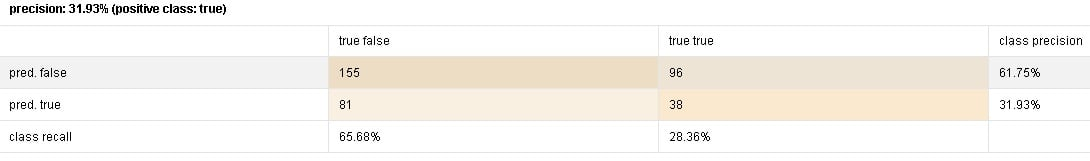So here is my question - Is this a:

1.) "Sometimes this happens when you try and forecast unseen data" kind of issue or is it a
2.) "You're a madman to take times series data and let Auto Model have its way with it" type of issue?

I.E. Is the notion that one can use Auto Model in conjunction with properly processed time series data fundamentally flawed?

Thanks, Noel
• Member Posts: 31Contributor II
edited May 4
Another possibility worth mentioning:
3.) "Apply Model" isn't the right operator for use with time series data in this situation
• Administrator, Moderator, Employee, RapidMiner Certified Analyst, RapidMiner Certified Expert, Community Manager, RMResearcher, Member, University Professor Posts: 1,619RM Founder
Hi,
I.E. Is the notion that one can use Auto Model in conjunction with properly processed time series data fundamentally flawed?
No, I don't think so.  But it is incredibly important that both data sets have been processes in exactly the same way since otherwise unexpected things can happen.  This could be the case.  One easy way of testing this is to include your test data with a missing label to original data fed into Auto Model.  It will then create the predictions for those rows (same order as in the input data) similar to the internally used hold-out set.  You can export those predictions, join the true labels back to the data, and calculate the performance again.  Is it better and more in line with what AM has estimated?  If yes, I would bet that there is something off in the preprocessing part...  However, if you test this as described above and it is still terrible, it could in theory be of the 'sometimes this happens kind' given that the standard deviation is pretty high with >9%...
Anyway, that's the first thing I would try...
Hope this helps,
Ingo
• Administrator, Moderator, Employee, RapidMiner Certified Analyst, RapidMiner Certified Expert, Community Manager, RMResearcher, Member, University Professor Posts: 1,619RM Founder
I just realized another thing though: is your test set from a later point in time than your training data?  Auto Model does not use proper backtesting (it would for a time series variant, but the current AM does not know anything specific about time series).  That means that there is some information leakage introduced by a random-hold-out sample which could lead to the worse result as well..
Cheers,
Ingo
• Member Posts: 31Contributor II
@IngoRM
Thank you very much for the reply.
I've been trying to complete the "first thing to try" you suggested above, but I'm having difficulty joining the "true" labels back onto the data. Auto Model explodes the index dates: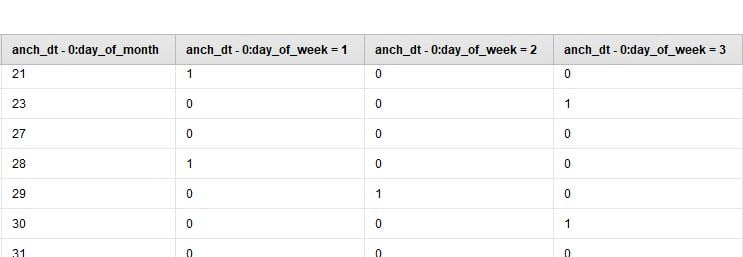I tried reconstituting the dates (but there isn't a date[year,month,day] function) as well as some other join attributes with no luck.

Would it be possible for someone at RapidMiner HQ to determine whether or not time series data works with Auto Model? If it does, you have yet another use case for Auto Model. If it doesn't, I can stop bashing my head against the wall and move onto another approach.

Best, Noel

( @varunm1 @CraigBostonUSA )
• Member Posts: 563Unicorn
Hello @Noel

I am not sure if there is anyone better than @IngoRM to clarify about automodel. From his response I can observe that automodel cannot distinguish time series.

Thanks
• Member Posts: 31Contributor II
OK, @varunm1, understood. I wasn't sure how to interpret both replies.

I wonder if they'll make a time series variant of Auto Model? ( @IngoRM )

Thanks again,
Noel
• Administrator, Moderator, Employee, RapidMiner Certified Analyst, RapidMiner Certified Expert, Community Manager, RMResearcher, Member, University Professor Posts: 1,619RM Founder
Yeah, sorry, I should have been clearer:
1. While AM can handle time series predictions if the data has been properly preprocessed before (e.g. with a windowing approach),...
2. ...the validation is not following the standard approach of backtesting, i.e. only train the model on older data than it is applied on.  This is likely the reason why the performances estimated by AM are too optimistic in your case.

I wonder if they'll make a time series variant of Auto Model? ( @IngoRM )
Yes, in fact we have this on our roadmap.  There is another bigger project we want to finish before in the AM world (I am not allowed to share the details yet - but the first part of the project name rhymes with "Bottle Enjoyment" and the second part of the project name rhymes with "Bottle Entanglement" - ok, the latter is a bit of a stretch...)
Cheers,
Ingo
• 12Administrator, Moderator, Employee, RapidMiner Certified Analyst, Community Manager, Member, University Professor, PM Moderator Posts: 2,351Community Manager

but the first part of the project name rhymes with "Bottle Enjoyment" and the second part of the project name rhymes with "Bottle Entanglement" - ok, the latter is a bit of a stretch...)

really @IngoRM??? 🤦‍♂️
• Member Posts: 31Contributor II
Thanks, @IngoRM - I can't wait to try the time series-enabled Auto Model... and find out the answers to the riddle.
• Member Posts: 31Contributor II
@IngoRM ( @varunm1 , @sgenzer ) -

(At @hughesfleming68 ;'s suggestion,) I've been working my way through Marcos López de Prado's book, "Advances in Financial Machine Learning". In it, López de Prado mentions "leakage" that is possible using Cross Validation with time series data. Is that the same "information leakage" you mentioned above with respect to Auto Model use and time series data?
Should folks working with time series data be using a different validation method (such as Forecast Validation)? López de Prado suggests a few alternatives to CV (which do not appear trivial in implement) and I'm hoping there is a standard validation operator in RM that I can use in place of CV.

Thank you, again, for your help. Best, Noel

• Administrator, Moderator, Employee, RapidMiner Certified Analyst, RapidMiner Certified Expert, Community Manager, RMResearcher, Member, University Professor Posts: 1,619RM Founder
Hi @Noel
That is exactly the type of leakage I was referring to.  Without knowing the details, I think this is likely the reason why AM shows better performance estimations since it does not take into account the nature of time in the current state.
Yes, there is an operator called "Forecast Validation" which should take care of this.
Cheers,
Ingo
• Member Posts: 31Contributor II
Hi @varunm1 -

Luckily, you seem to be following along, so I'll ask you this questionAs @IngoRM suggested, I'm trying to use Forecast Validation in my process instead of Cross Validation, but I keep getting a "does not have a label attribute" error: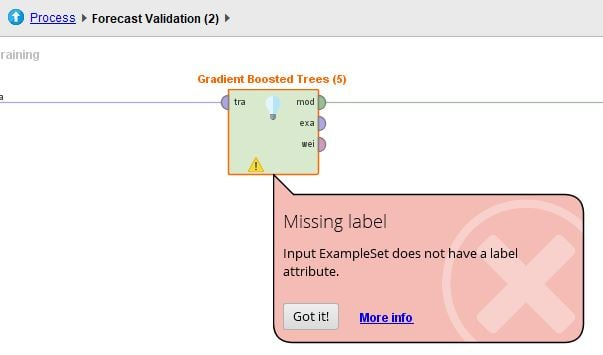I can't figure out what's wrong. I set the role and everything looks fine at the break point: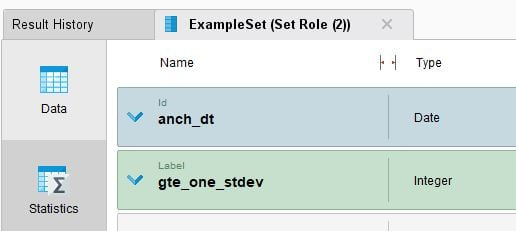Any help would be, as always, most appreciated. I've attached an xl file and sample process.

Thanks!
• Member Posts: 563Unicorn
edited May 13
Hello @Noel

I found the issue but I don't know why this happened as I didnot use this operator earlier. I can see that the input going into the GBT model is shown below, and this doesn't make sense to me. You can see this doesn't have a label (your earlier label role is removed automatically) and also there are no other attributes. I think you might be choosing something wrong using forecast validation. @hughesfleming68 can suggest you on this.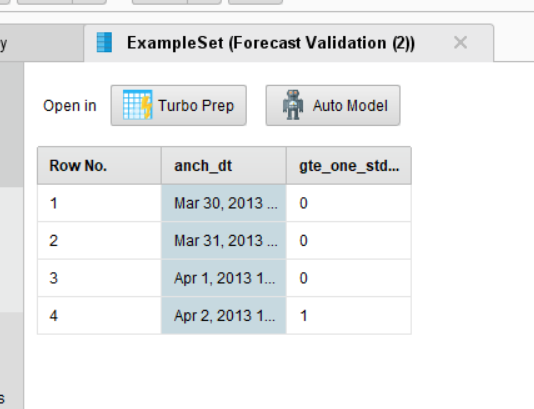Thank you
• Member, KB Contributor Posts: 128Guru
Hi every body
It is the old problem which I saw that for several time.
please be careful about using a data for several time or also the name of each column is really important.
I had a column with two words and my RM could not understand it and also I have to rename my column and rename the data in order to RM understand it. and also be careful about repository. do not repeat a data there
Regards
mbs
• Employee, RapidMiner Certified Analyst, RapidMiner Certified Expert, RMResearcher, Member Posts: 92RM Research
Concerning the error in the Forecast Validation: Please note that this is a validation of Time Series Forecast models, and currently cannot handle standard machine learning models (e.g. the Gradient Boosted Tree). You can either use a Forecast model on the time series data inside the Forecast Validation (currently supported are Arima, Holt-Winters and with 9.3 Default Forecast and Function and Seasonal Forecast).
If you want to validate a standard ml model on your time dependent data, you should use the Sliding Window Validation of the Value Series extension (install over marketplace). You may need to apply Windowing before training and testing the model. We are still improving the time series functionality in RapidMiner with every update and the overhaul of the Sliding Window Validation of the old value series extension, as well as a way to use standard ml models in Forecast Validation is on the road map.

Hopes this helps
Best regards
Fabian
• Member Posts: 31Contributor II
@tftemme - Thanks, Fabian.

I'm a little confused by the Sliding Window Validation terminology. It seems like the "training window width" and "test window width" are distinct from the structure of the data created via the "Windowing" or "Process Windows" operators,
In my process, I've windowed the data to have a window size of 5 periods, an offset of 3 periods, and a horizon size of 1 period. The data is a series of observations over 1500 periods long.
So,
period 1
period 2
period 3
...
period 20
becomes:
window 1 = per 1, per 2, per 3, per 4, per 5
window 2 = per 2, per 3, per 4, per 5, per 6
window 3 = per 3, per 4, per 5, per 6, per 7
...
window 16 = per 16, per 17, per 18, per 19, per 20

For Sliding Window Validation, it seems like we're specifying the number of windows (in the sense above) to use to train followed by the number of windows to use for testing. Is that right? If that is the case, what should the relationship be between the time frame (window) for training and the time frame (window) for testing? What should the relationship be between these time frames/windows and the total number of periods in the time series?

Thanks,
Noel

• Member Posts: 31Contributor II
@tftemme ( @varunm1 / @hughesfleming68 ) -

I have no idea how to size the training and testing windows for Sliding Window Validation. I tried the default 100 for both but it seems to take forever to run relative to Cross Validation. And the results are comparatively horrible.

I have roughly 1,500 periods of (daily) time series data and I've created windows each with 20 periods... what are reasonable validation window sizes? Is there any detailed documentation (or better yet examples of use) for the Sliding Window Validation operator?

With "plain" windowing, you have a time frame containing some number of periods, a horizon (the label to be predicted), and a horizon offset (number of periods from the end of the window to the horizon). With this validation operator, the training window is described as "Number of examples in the window which is used for training", horizon as "Increment from last training to first testing example", and testing window as "Number of examples which are used for testing (following after 'horizon' examples after the training window end)".

This "horizon" sounds like the "offset" concept with plain windowing. Should the validation windows be the same size? When would you want to use the cumulative training option and when would you not?

Thanks, Noel

• Member Posts: 31Contributor II
Attached are data and an example process.
• Employee, RapidMiner Certified Analyst, RapidMiner Certified Expert, RMResearcher, Member Posts: 92RM Research
Hi @Noel,

I will try an explanation. I think most of it you already get correct, and its only some smaller things which seems to be the problem.

So the approach you would follow to built and validate a standard ml model for forecasting time series data is
1) Use Windowing to convert your time series forecast problem in a standard ML problem
- Time Series Forecast problem: You have (multiple) time series (in form of Attributes) and you want to forecast future values (aka. examples for future timestamps)
- Standard ML problem: You have (independent) examples with attributes and you want to predict the value of a specific attribute (aka the label attribute)
=> After applying windowing you have (technically) an ExampleSet with attributes (the last values in the window) and a label (the horizon). So you can train any ML model on it
2) The problem is that it is only technically the same situation. The Examples are not independent of each other. Therefore you use Sliding Window Validation on your windowed ExampleSet to validate the performance of your ML model. The Sliding Window Validation only divides this ExampleSet into training and test sets:
First Iteration:
- The training set are the Examples number [ 0 , n ] of the input ExampleSet (n: training window width).
- Training size in Sliding Window Validation: n
- Training size in X-fold Cross Validation: (x-1) / x * total number of Examples
- The test set are the Examples number [ n + h , n + h + t ] of the input ExampleSet (h: horizon ; t: test window width).
- Test size in Sliding Window Validation: t
- Test size in X-fold Cross Validation: 1 / x * total number of Examples
Second Iteration:
- The training set is moved s Examples => [ s , s + n ] (s: training window step size)
- If s = -1 ; s will be set to t; this assures that all test sets are independent of each other
- The test set is also moved (cause it is starting h values behind the actual training set: [ s + n + h , s + n + h + t ]
i-th Iteration:
- Training Set: [ (i-1) * s ,  (i-1)*s + n ]
- Test Set: [ (i-1)*s + n + h , (i-1)*s + n + h + t ]
The Sliding Window Validation stops if (i-1)*s + n + h + t is larger than total number of Examples.
Hence number of iterations is: 1 + (N - n - h - t) / s

Ok, this is the general approach. Back to your example:
You should choose the training window width to have a "suitable" number of Examples for training the ML model and as well the test window width to have a "suitable" number of Examples to test the model. For the horizon you just can take 1, cause your next Example already have as a label the time series values 5 days in the future (created by the Windowing). Last you should choose the training window step size, so that you have a "good" number of iterations (and you should also take into account, if the training window step size is smaller than test window width, that your test sets will have overlapping examples (hence the -1 option exist).
Here I wrote "suitable" and "good" cause this depends always on your data, the model etc.
So here are the settings you had and my suggestions to improve on them (keep in mind that you have 1802 Examples after Windowing):
n = 100  => maybe a bit less Examples to train the GBT => I would use 1300
t = 100 => large enough to get a proper performance, small enough that you have a decent number of iterations => keep this
h = 4 => As explained, just go with 1
s = 1 => Only moving training set 1 Example, so you have overlapping test windows and you have 1 +  (1802 - 100 - 4 - 100 ) / 1 = 1599 iterations, which explains the long runtime => I would go with -1 so same as t). Hence 1 +  (1802 - 1300 - 1 - 100 ) / 100 = 5 number of iterations

Hopes this explains the situation (Explanation is getting longer. I should keep this for the docu, once we start to include the Sliding Window Validation in the new time series extension).

Best regards,
Fabian
• Member Posts: 31Contributor II
Fabian / @tftemme -

Thanks for taking the time to spell all that out. Your explanation cleared up a lot for me and I really appreciate it. I have a few followup questions, thoughWith respect to the Sliding Window Validation operator:
You say that the training and test window sizes should be "suitable". Are those parameters that it would make sense to optimize?
I see why setting the step size to -1 is the right thing for me right now, but are there situations where one would want overlapping windows?
When would it make sense to use the cumulative training option?

Regarding horizons:

I'd like to see if I can forecast more than one period into the future. My data contains labels indicating when the target attribute made a one standard deviation move (or more) over the previous five periods. Prior to switching to the Sliding Window Validation operator, I was experimenting with different window widths (I'm talking about "regular" windowing now) to see which had the best precision and recall. After each window, I had an offset of four and a horizon of one. How do I replicate this exercise with the Sliding Window Validation operator (with just a single horizon concept)? When I was using Cross Validation, it seemed like I was getting pretty good results: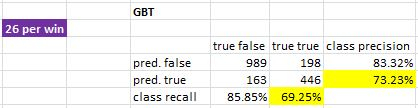Should I see similar performance with Sliding Window Validation?

Finally, you mentioned that you would use ~1,300 periods of data to train a GBT...
How did you arrive at that number? Would you use a different length for SVM or RF?
I used all the history I had while reserving the last year for out of sample testing. I gather more is not necessarily better?

Thanks again!
-Noel

( @varunm1 , @hughesfleming68 )
• Employee, RapidMiner Certified Analyst, RapidMiner Certified Expert, RMResearcher, Member Posts: 92RM Research
Hi @Noel ,

Choosing a "suitable" training window size and test window size would be the same questions of choosing the number of folds in the Cross Validation if you have a small number of Examples in total. There is always a trade off between enough Examples to proper train the model and enough Examples to proper test your model. For Cross Validation if you choose a larger number of folds, the training sets will increase, the test sets will decrease in size (but obviously more testing iterations to average the performance). In Sliding Window Validation it is the same, more training Examples leads to less for testing or less iterations to average the performance.
As these are only parameters how the validation is executed and the final model is built on all Examples, it does not make sense to optimize them (would have the same meaning of optimizing the number of folds of a Cross Valdiation).

When to go with overlapping windows? As a rule of thumb I would always try to not have overlapping test windows, to be completely sure that no bias is included in the performance evaluation. But if you have a smaller number of events you maybe want to go with overlapping windows to have a proper number of iterations in your validation, so that the averaging of the performances is statistically speaking reasonable.

I think you would use the cumulative option if you wants to validate the situation when you continuously add data to the training of your model. I did not used this yet.

Sliding Window Validation and the Windowing operator still have different wording: So horizon offset in the Windowing should be the same as horizon in Sliding Window Validation, while the horizon size in Windowing is the same as the test window width in Sliding Window Validation.
Keep in mind that the operation are still different. While Windowing converts the time series data to a windowed ExampleSet the Sliding Window Validation splits the input ExampleSet into two different ExampleSets.

I think for Optimizing the window size of the windowing you would need roughly the following setup:

```<process version="9.2.001">
<context>
<input/>
<output/>
<macros/>
</context>
<operator activated="true" class="process" compatibility="9.2.001" expanded="true" name="Process">
<parameter key="logverbosity" value="init"/>
<parameter key="random_seed" value="2001"/>
<parameter key="send_mail" value="never"/>
<parameter key="process_duration_for_mail" value="30"/>
<parameter key="encoding" value="SYSTEM"/>
<process expanded="true">
<operator activated="true" class="retrieve" compatibility="9.2.001" expanded="true" height="68" name="Retrieve Prices of Gas Station" width="90" x="246" y="34">
<parameter key="repository_entry" value="//Samples/Time Series/data sets/Prices of Gas Station"/>
</operator>
<operator activated="true" class="concurrency:optimize_parameters_grid" compatibility="9.2.001" expanded="true" height="124" name="Optimize Parameters (Grid)" width="90" x="447" y="34">
<list key="parameters">
<parameter key="Windowing.window_size" value="[10;50;4;linear]"/>
</list>
<parameter key="error_handling" value="fail on error"/>
<parameter key="log_performance" value="true"/>
<parameter key="log_all_criteria" value="false"/>
<parameter key="synchronize" value="false"/>
<parameter key="enable_parallel_execution" value="true"/>
<process expanded="true">
<operator activated="true" class="series:sliding_window_validation" compatibility="7.4.000" expanded="true" height="124" name="Validation" width="90" x="179" y="34">
<parameter key="create_complete_model" value="false"/>
<parameter key="training_window_width" value="6000"/>
<parameter key="training_window_step_size" value="-1"/>
<parameter key="test_window_width" value="1000"/>
<parameter key="horizon" value="1"/>
<parameter key="cumulative_training" value="false"/>
<parameter key="average_performances_only" value="true"/>
<process expanded="true">
<operator activated="true" class="time_series:windowing" compatibility="9.3.000-SNAPSHOT" expanded="true" height="82" name="Windowing" width="90" x="112" y="34">
<parameter key="attribute_filter_type" value="single"/>
<parameter key="attribute" value="gas price / euro (times 1000)"/>
<parameter key="attributes" value=""/>
<parameter key="use_except_expression" value="false"/>
<parameter key="value_type" value="nominal"/>
<parameter key="use_value_type_exception" value="false"/>
<parameter key="except_value_type" value="time"/>
<parameter key="block_type" value="single_value"/>
<parameter key="use_block_type_exception" value="false"/>
<parameter key="except_block_type" value="value_matrix_row_start"/>
<parameter key="invert_selection" value="false"/>
<parameter key="include_special_attributes" value="false"/>
<parameter key="has_indices" value="true"/>
<parameter key="indices_attribute" value="date"/>
<parameter key="window_size" value="20"/>
<parameter key="no_overlapping_windows" value="false"/>
<parameter key="step_size" value="1"/>
<parameter key="create_horizon_(labels)" value="true"/>
<parameter key="horizon_attribute" value="gas price / euro (times 1000)"/>
<parameter key="horizon_size" value="1"/>
<parameter key="horizon_offset" value="4"/>
</operator>
<operator activated="true" class="concurrency:parallel_decision_tree" compatibility="9.2.001" expanded="true" height="103" name="Decision Tree" width="90" x="246" y="34">
<parameter key="criterion" value="least_square"/>
<parameter key="maximal_depth" value="10"/>
<parameter key="apply_pruning" value="true"/>
<parameter key="confidence" value="0.1"/>
<parameter key="apply_prepruning" value="true"/>
<parameter key="minimal_gain" value="0.01"/>
<parameter key="minimal_leaf_size" value="2"/>
<parameter key="minimal_size_for_split" value="4"/>
<parameter key="number_of_prepruning_alternatives" value="3"/>
</operator>
<connect from_port="training" to_op="Windowing" to_port="example set"/>
<connect from_op="Windowing" from_port="windowed example set" to_op="Decision Tree" to_port="training set"/>
<connect from_op="Decision Tree" from_port="model" to_port="model"/>
<portSpacing port="source_training" spacing="0"/>
<portSpacing port="sink_model" spacing="0"/>
<portSpacing port="sink_through 1" spacing="0"/>
</process>
<process expanded="true">
<operator activated="true" class="clone_parameters" compatibility="9.2.001" expanded="true" height="82" name="Clone Parameters" width="90" x="45" y="85">
<list key="name_map">
<parameter key="Windowing.window_size" value="Windowing (2).window_size"/>
</list>
</operator>
<operator activated="true" class="time_series:windowing" compatibility="9.3.000-SNAPSHOT" expanded="true" height="82" name="Windowing (2)" width="90" x="179" y="85">
<parameter key="attribute_filter_type" value="single"/>
<parameter key="attribute" value="gas price / euro (times 1000)"/>
<parameter key="attributes" value=""/>
<parameter key="use_except_expression" value="false"/>
<parameter key="value_type" value="nominal"/>
<parameter key="use_value_type_exception" value="false"/>
<parameter key="except_value_type" value="time"/>
<parameter key="block_type" value="single_value"/>
<parameter key="use_block_type_exception" value="false"/>
<parameter key="except_block_type" value="value_matrix_row_start"/>
<parameter key="invert_selection" value="false"/>
<parameter key="include_special_attributes" value="false"/>
<parameter key="has_indices" value="true"/>
<parameter key="indices_attribute" value="date"/>
<parameter key="window_size" value="20"/>
<parameter key="no_overlapping_windows" value="false"/>
<parameter key="step_size" value="1"/>
<parameter key="create_horizon_(labels)" value="true"/>
<parameter key="horizon_attribute" value="gas price / euro (times 1000)"/>
<parameter key="horizon_size" value="1"/>
<parameter key="horizon_offset" value="4"/>
</operator>
<operator activated="true" class="apply_model" compatibility="9.2.001" expanded="true" height="82" name="Apply Model" width="90" x="313" y="34">
<list key="application_parameters"/>
<parameter key="create_view" value="false"/>
</operator>
<operator activated="true" class="performance_regression" compatibility="9.2.001" expanded="true" height="82" name="Performance" width="90" x="447" y="34">
<parameter key="main_criterion" value="first"/>
<parameter key="root_mean_squared_error" value="true"/>
<parameter key="absolute_error" value="false"/>
<parameter key="relative_error" value="false"/>
<parameter key="relative_error_lenient" value="false"/>
<parameter key="relative_error_strict" value="false"/>
<parameter key="normalized_absolute_error" value="false"/>
<parameter key="root_relative_squared_error" value="false"/>
<parameter key="squared_error" value="false"/>
<parameter key="correlation" value="false"/>
<parameter key="squared_correlation" value="false"/>
<parameter key="prediction_average" value="false"/>
<parameter key="spearman_rho" value="false"/>
<parameter key="kendall_tau" value="false"/>
<parameter key="skip_undefined_labels" value="true"/>
<parameter key="use_example_weights" value="true"/>
</operator>
<connect from_port="model" to_op="Apply Model" to_port="model"/>
<connect from_port="test set" to_op="Clone Parameters" to_port="through 1"/>
<connect from_op="Clone Parameters" from_port="through 1" to_op="Windowing (2)" to_port="example set"/>
<connect from_op="Windowing (2)" from_port="windowed example set" to_op="Apply Model" to_port="unlabelled data"/>
<connect from_op="Apply Model" from_port="labelled data" to_op="Performance" to_port="labelled data"/>
<connect from_op="Performance" from_port="performance" to_port="averagable 1"/>
<portSpacing port="source_model" spacing="0"/>
<portSpacing port="source_test set" spacing="0"/>
<portSpacing port="source_through 1" spacing="0"/>
<portSpacing port="sink_averagable 1" spacing="0"/>
<portSpacing port="sink_averagable 2" spacing="0"/>
</process>
</operator>
<connect from_port="input 1" to_op="Validation" to_port="training"/>
<connect from_op="Validation" from_port="model" to_port="model"/>
<connect from_op="Validation" from_port="averagable 1" to_port="performance"/>
<portSpacing port="source_input 1" spacing="0"/>
<portSpacing port="source_input 2" spacing="0"/>
<portSpacing port="sink_performance" spacing="0"/>
<portSpacing port="sink_model" spacing="0"/>
<portSpacing port="sink_output 1" spacing="0"/>
</process>
</operator>
<connect from_op="Retrieve Prices of Gas Station" from_port="output" to_op="Optimize Parameters (Grid)" to_port="input 1"/>
<connect from_op="Optimize Parameters (Grid)" from_port="performance" to_port="result 2"/>
<connect from_op="Optimize Parameters (Grid)" from_port="model" to_port="result 3"/>
<connect from_op="Optimize Parameters (Grid)" from_port="parameter set" to_port="result 1"/>
<portSpacing port="source_input 1" spacing="0"/>
<portSpacing port="sink_result 1" spacing="0"/>
<portSpacing port="sink_result 2" spacing="0"/>
<portSpacing port="sink_result 3" spacing="0"/>
<portSpacing port="sink_result 4" spacing="0"/>
</process>
</operator>
</process>
```
I moved the Windowing inside the Sliding Window Validation (both in training and testing). I used Clone Parameters to make sure that the window size of the second Windowing operator is the same as the first one (and realized, that Windowing needs a preprocessing model ;-)

Now I can use an Optimize operator to optimize the window size parameter.

Whether the results of Cross Validation and Sliding Window Validation differ depends on your data and how much of the time-dependent bias is "leaking" in the Cross Validation.

Finally, how I came up with the values:
So these are all rules of thumb:
a) I want to have 5 iterations (normally I plan for 5-10) for validation, so that the average of the performance values of the iterations is reasonable
b) I want to have 100 test Examples (if my total number , so that the performance evaluation for one iteration is reasonable)
c) I don't want to have overlapping test windows (=> step size =1)
d) Hence n = N - (I - 1) * s - h - t ; N: Total number of events; I: number of ierations; s: stepsize; h: horizon; t: test window width
n = 1802 - (5-1) * 100 - 1 - 100 = 1301 (ok rounded down to 1300)

I think I would use the same number of training events independently of the model. Again this is only for validation, final model is built on all Examples

Best regards,
Fabian

• Member Posts: 31Contributor II
edited May 17
Fabian ( @tftemme ) -
Thank you, again, for taking time to write out those explanations.

It is clear that I need to invest more time learning about validating time series data before I ask you any more questions about RapidMiner's time series operators. Based on my reading, using alternate validation schemes is critical. Marcos López de Prado's recent book takes a strong stance: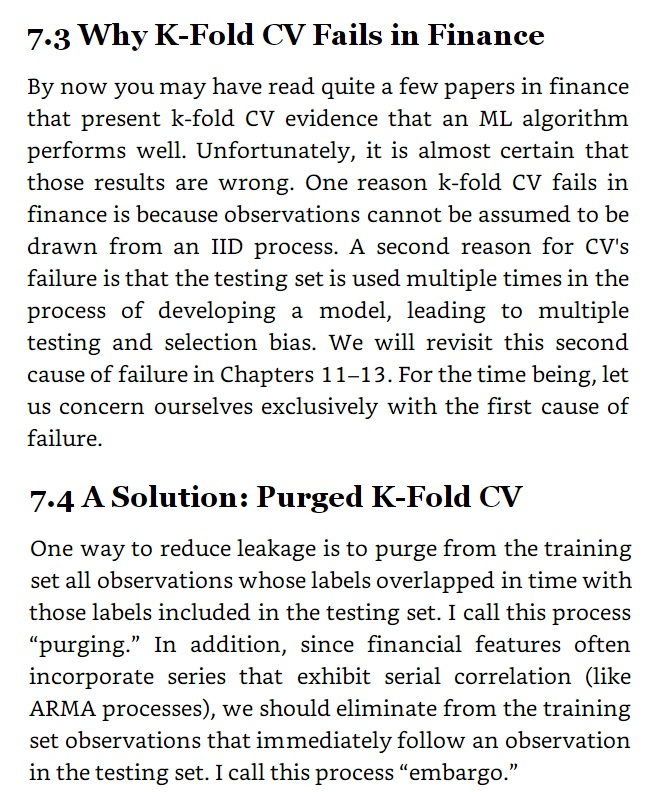and other folks have things to say as well:

( @IngoRM @hughesfleming68